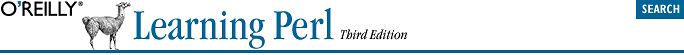home | O'Reilly's CD bookshelfs | FreeBSD | Linux | Cisco | Cisco Exam## 17.2. Picking Items from a List with grep

Sometimes you'll want only certain items from a list. Maybe it's only the odd numbers selected from a list of numbers, or maybe it's only the lines mentioning Fred from a file of text. As we'll see in this section, picking some items from a list can be done simply with the grep operator.

Let's try that first one and get the odd numbers from a large list of numbers. We don't need anything new to do that:

```my @odd_numbers;

foreach (1..1000) {
push @odd_numbers, \$_ if \$_ % 2;
}```

That code uses the modulus operator (%), which we saw in Chapter 2, "Scalar Data". If a number is even, that number "mod two" gives zero, which is false. But an odd number will give one; since that's true, only the odd numbers will be pushed onto the array.

Now, there's nothing wrong with that code as it stands -- except that it's a little longer to write and slower to run than it might be, since Perl provides the grep operator:

`my @odd_numbers = grep { \$_ % 2 } 1..1000;`

That line gets a list of 500 odd numbers in one quick line of code. How does it work? The first argument to grep is a block that uses \$_ as a placeholder for each item in the list, and returns a Boolean (true/false) value. The remaining arguments are the list of items to search through. The grep operator will evaluate the expression once for each item in the list, much as our original foreach loop did. For the ones where the last expression of the block returns a true value, that element is included in the list that results from grep.

While the grep is running, \$_ is aliased to one element of the list after another. We've seen this behavior before, in the foreach loop. It's generally a bad idea to modify \$_ inside the grep expression, because this will damage the original data.

`my @matching_lines = grep { /\bfred\b/i } <FILE>;`

There's a simpler syntax for grep, too. If all you need for the selector is a simple expression (rather than a whole block), you can just use that expression, followed by a comma, in place of the block. Here's the simpler way to write that latest example:

`my @matching_lines = grep /\bfred\b/i, <FILE>;`